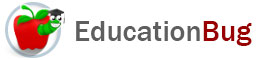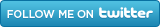PUBLIC SCHOOLS PRIVATE SCHOOLS SCHOOL DISTRICTS COLLEGES PUBLIC LIBRARIES JOBS BLOG RESOURCES
Career Education
Education Choices
Education Costs
Education History and General Information
Education Issues
Education Resources
Family Involvement in Education
Students w Special Needs
Subject Information and Homework Help
Teaching and Learning
Testing
By Types of Schools
By Specific Audiences
GeneralMath Symbols

That math problems in words are labeled â€œword problemsâ€ is  reminder to us that it is typical for mathematical communication to be made without words, so it's critical to understand  the meanings of math symbols. Read on for more information about common math symbols.

Math Symbols: Numerals

Although you wouldnâ€™t know it from looking in the dictionary, the items we write in math containing 0, 1, 2, 3, 4, 5, 6, 7, 8, and 9 are not numbers. They are numerals which represent numbers. A number is actually an abstract unit of a mathematical system.

The system using the 10 digits mentioned above is called Arabic numerals. Combined with , which is used to separate groups of three digits from each other, - which indicates a negative number, and . the decimal point, they are used to convey the rational numbers.

The Roman numerals is another system for conveying numbers. It is a notation system without a zero and is derived from an ancient Roman system. The basic set of symbols used are some of the capital letters:

I           V            X            L            C            D            M

Numbers that are not rational numbers require different symbols to express them. Two of the most common are pi, for which the math symbol is Î  and the square root of 2, for which the math symbol is âˆš2

Math Symbols: Operations

An important aspect of math is manipulating numbers in various ways. A great deal of what is done with numbers falls under the category of operations. The basic mathematical operations can be conveyed in words or symbols. When writing equations, symbols are used.

• Subtraction is shown with the â€œminus signâ€  -  the same symbol that is used to indicate negative numbers, for example, x - y  (For more about subtraction, see the article â€œSubtraction.â€)
• Division is shown either by the â€œdivision signâ€  Â¸ for example, x Â¸ y or a â€œfraction slashâ€  â„    for example x â„ y (For more about division, see the article â€œDivision.â€)
• There are multiple ways to show the multiplication operation. The symbol that is usually used to begin with is x  for example,  2  x  3 Other symbols to convey multiplication include:

a dot  âˆ™ called a â€œbullet operatorâ€ or â€œmiddle dot,â€ for example x âˆ™ y

an asterisk * in programming language,

a superscript numeral like this xÂ² for an exponent,

and - in algebra - juxtaposition, either with or without parentheses, for example

xy or x(y) (For more about multiplication, see the article â€œMultiplication.â€)

• There is another use of parentheses that is important to mention. When an equation or inequality has multiple operations, one doesnâ€™t just proceed from left to right in solving it:  there is a standard order of operations to follow. One begins with exponents, then multiplication and division, then addition and subtraction. However, parentheses are used to supersede the standard order of operations and make sure that a certain operation or group of operations is performed prior to others, and if you take the first letter of each, you get PEMDAS. The mnemonic â€œPlease excuse my dear Aunt Sallyâ€ is used by many as they are learning the order of operations.

Math Symbols: Equalities and Inequalities

Math instruction usually begins with solving equations. The â€œequal signâ€  = is the symbol used between the two equivalent quantities. Later, inequalities are introduced, with a new set of math symbols to convey the various possible relationships between the quantities represented by each side. Here they are:

• not equal to is represented by this symbol: Â¹
• almost equal to is shown by this symbol: Â»
• less than is shown like this: <
• greater than is conveyed like this: >
• less than or equal to is represented like this: â‰¤
• greater than or equal to is represented like this:  Â³
• approximately equal to is shown by this symbol: @

Math Symbols: Set Theory

In set theory, the relationships between items and groups is shown by a special set of math symbols. Here are some of the basic ones:

• groups that intersect Ãˆ
• groups that do not intersect  âˆ©
• is an element of âˆˆ
• is not an element of Ã
• is a subset of ÃŒ
• is not a subset of Ã‹